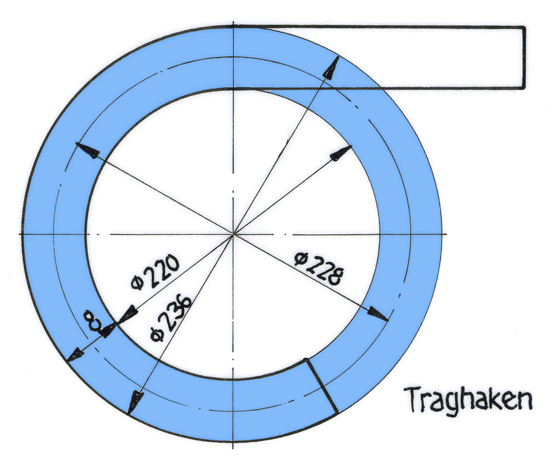# How to calculate the pipe length for bending

## Calculation of stretched lengths19.11.2010, 09:34

Before producing a bent part, you have to know its "stretched" length. It is determined using the neutral fiber. If it is a straight rod with a rectangular, round or otherwise symmetrical cross-section, then the neutral fiber lies in the middle of the cross-section. In the case of other cross-sectional shapes, the position of the neutral fiber must first be determined.

1st year of training

### Calculate straight lengths

Before producing bent parts, their "stretched" length L must be calculated. It is the length to be sawn off and corresponds to the neutral grain of the part. With symmetrical cross-sections (round, rectangular cross-section), the neutral fiber lies exactly in the middle between the outer and inner bending radius. For very small bending radii, the calculation must be carried out with a compensation factor (see below).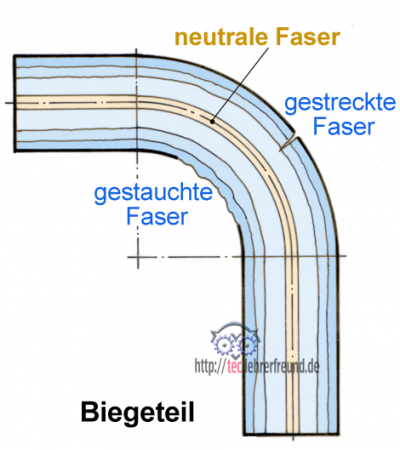In technical mechanics, a neutral fiber is the line of a bending cross-section, the length of which does not change during a bending process. The fibers lying further outside are stretched during bending, while those further inside are compressed. The stretched fibers run the risk of bending cracks due to excessive stress on the workpiece or if the bending radius is too small.

The term »fiber« is based on the idea that the material is built up in individual layers, as they actually arise in several rolling processes.

Calculation example Carrying hook, Flat steel 8 x 12

Calculate the stretched length.

The support hook consists of a straight section l1 and an open arc l2. When calculating the circular arc, the neutral fiber in the center of the cross-section must be used: dm = 220 mm + 2 • 4 mm = 228 mm.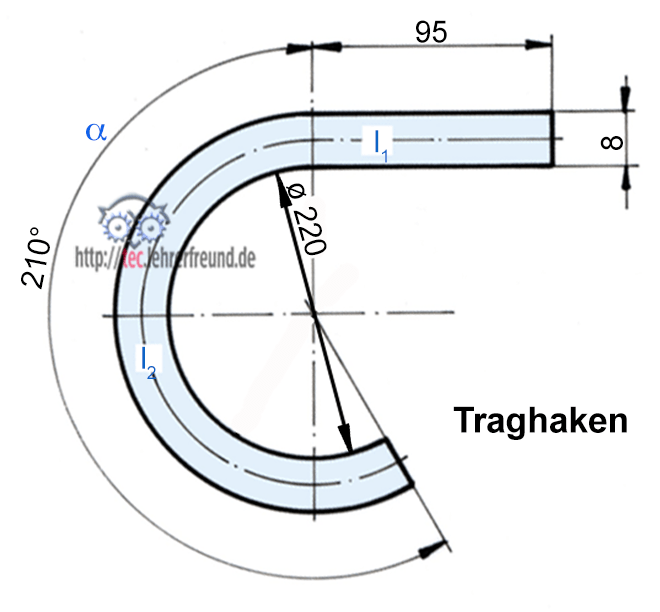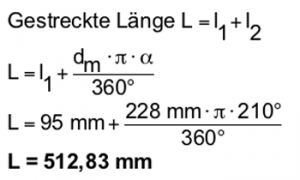Calculation example Pipe clamp, Round steel ø12

Calculate the stretched length.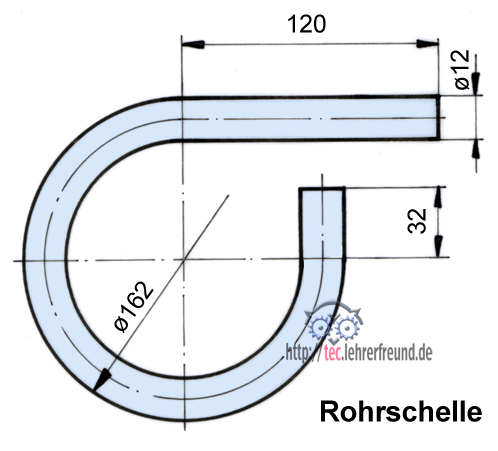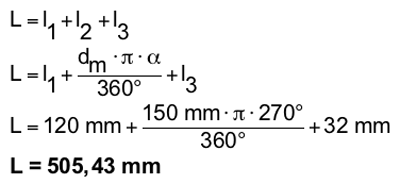Calculation example Handle, Round steel ø5

Calculate the stretched length.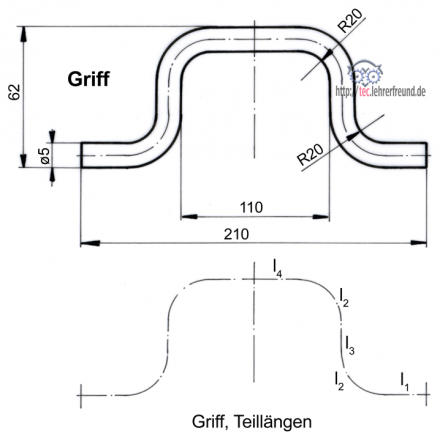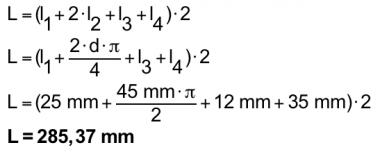In the case of bending radii, the radius R of which is less than 5 times the sheet thickness (R <5 • s), the neutral fiber is shifted due to additional material deformations. Then the length of the neutral fiber needs to be corrected. The Compensation value for it is v; you can find it in tables. The following formula is then used for 90 ° bending angles:

L = l1 + l2 + l3 + ... - n • v (n = number of bending points on the workpiece)

Table of compensation values: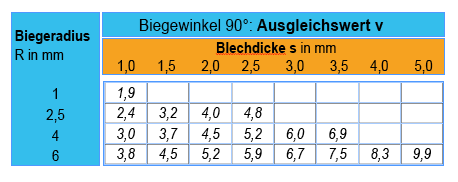Calculation example Handle (above) with radii reduced to R = 6 mm, four bending points:

L = (l1 + 2 • l2 + l3 + l4) • 2 - 4 • v
L = (l1 + 2 • d • 3.14: 4 + l3 + l4) • 2 - 4 • v
L = 39 mm + 17 mm • 3.14: 2 + 40 + 49) • 2 - 4 • 9.9 =

L = 269.8 mm

___________________________

Supplement comment sts